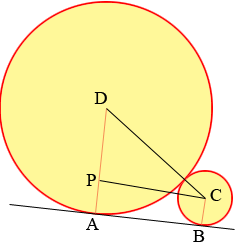SEARCH HOMEMath Central Quandaries & QueriesQuestion from Michele, a parent: A circle of radius 2 is externally tangent to a circle of radius 8, How do you find the length of their common tangent.Hi Michele,Are you looking for the length of the line segment AB?

Since the line AB is tangent to both circles angles DAB and ABC are right angles. From C the centre of the smaller circle construct a line parallel to AB and meeting DA at P. Triangle DPC is thus a right triangle. |DC| = 2 + 8 = 10, |DP| = 8 - 2 = 6. What is the length of PC?

PennyMath Central is supported by the University of Regina and The Pacific Institute for the Mathematical Sciences.